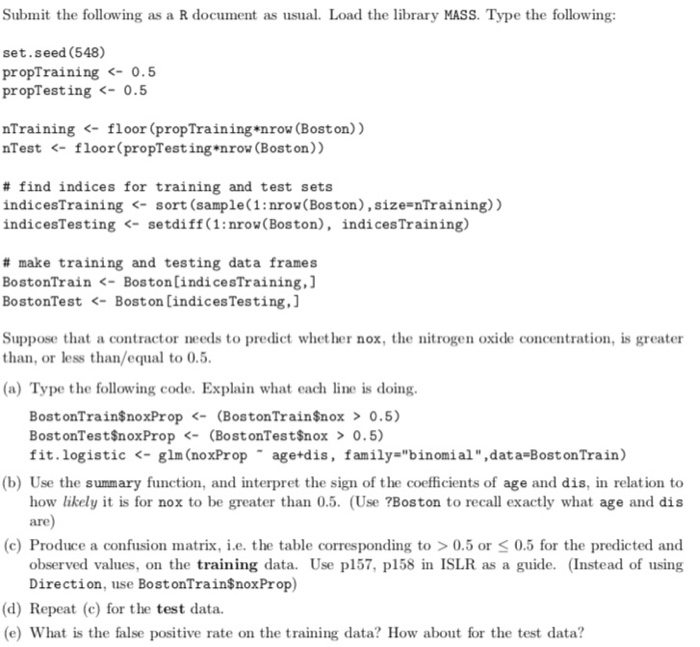Homework Help Question & Answers

Submit the following as a R document as usual. Load the library MASS. Type the following: set.see...

Code in R:Submit the following as a R document as usual. Load the library MASS. Type the following: set.seed (548) propTraining

library('MASS')
set.seed(548)
propTraining <- 0.5
propTesting <- 0.5nTraining <- floor(propTraining*nrow(Boston))
nTesting <- floor(propTesting*nrow(Boston))
nrow(Boston)
nTraining
nTesting

# find indices for training and test sets

indicesTraining <- sort(sample(1:nrow(Boston),size=nTraining))
indicesTesting <- setdiff(1:nrow(Boston),indicesTraining)

indicesTraining
indicesTesting

# make training and testing dataframe

BostonTrain <- Boston[indicesTraining,]
BostonTest <- Boston[indicesTesting,]

nrow(BostonTrain)
nrow(BostonTest)
(a)
BostonTrain\$noxProp <- (BostonTrain\$nox > 0.5) #Check the column 'nox' whether it is greater than 0.5 and return boolean TRUE/FALSE  and save it in new column named 'noxProp'

BostonTest\$noxProp <- (BostonTest\$nox > 0.5) #Check the column 'nox' whether it is greater than 0.5 and return boolean TRUE/FALSE and save it in new column named 'noxProp'

fit.logistic <- glm(noxProp~age+dis,family = "binomial",data=BostonTrain)

#fit logistic regression model, where independent variable Y is noxProp with value FALSE (0) or TRUE (1)

#and dependent variables X1,X2... are 'age' and 'dis'

(b)

> summary(fit.logistic)

Call:
glm(formula = noxProp ~ age + dis, family = "binomial", data = BostonTrain)

Deviance Residuals:
Min 1Q Median 3Q Max
-2.4833 -0.1914 0.1756 0.3052 2.6807

Coefficients:
Estimate Std. Error z value Pr(>|z|)
(Intercept) 0.62335 1.13932 0.547 0.584
age 0.05405 0.01138 4.751 2.02e-06 ***
dis -0.96741 0.17516 -5.523 3.33e-08 ***
---
Signif. codes: 0 ‘***’ 0.001 ‘**’ 0.01 ‘*’ 0.05 ‘.’ 0.1 ‘ ’ 1

(Dispersion parameter for binomial family taken to be 1)

Null deviance: 334.88 on 252 degrees of freedom
Residual deviance: 127.95 on 250 degrees of freedom
AIC: 133.95

Number of Fisher Scoring iterations: 6

Going through the summary, we can conclude that age and dis are both significant as value for both of these variables are much less than 0.05

logistic regression equation would be:

Y = 0.62335 +  0.05405 * age - 0.96741 * dis

This equation would calculate the the value of Y

going by the equation, we can conclude greater the value of dis there is more chance that nox would be less than 0.5

(c) confusion matrix on training data:

BostonTrain\$Predicted <- predict(fit.logistic,BostonTrain) # fitting the model on training data

table(BostonTrain\$noxProp, BostonTrain\$Predicted > 0.5)

FALSE TRUE
FALSE 84 11
TRUE 13 145

(d) Confusion matrix on test data :

BostonTest\$Predicted <- predict(fit.logistic,BostonTest)

table(BostonTest\$noxProp, BostonTest\$Predicted > 0.5)

FALSE TRUE
FALSE 84 13
TRUE 22 134

(e) False positive rate mean ratio of observation with were are actually false(0) but it has been categorized as true(1) by the model

so looking at the training data we can conclude : 11 observation were predicted as true which should actually be false

hence FPR = 11/(11+84) = 0.1157895

like wise for test data set

FPR = 13 / (13+ 84) = 0.1340206

Add Answer of: Submit the following as a R document as usual. Load the library MASS. Type the following: set.see...
More Homework Help Questions Additional questions in this topic.

• Per the guidelines set out in this document OR As per the guidelines set out in this document Which is correct

Need Online Homework Help?

Get FREE EXPERT Answers
WITHIN MINUTES
Related Questions